# Supervised Learning¶

In :
from ipypublish import nb_setup


Supervised Learning is one of the two major paradigms used to train Neural Networks, the other being Un-Supervised Learning. Supervised Learning is the easier problem to solve and historically appeared first with models such as the Perceptron. When progress in Supervised Learning stalled in the 80s and 90s due to the difficulties encountered in training DLNs with multiple Hidden Layers, researchers focused on Un-Supervised Learning and came up with systems such the Boltzmann Machine and its multiple Hidden Layer counterpart called Deep Belief Networks, see Le Roux and Bengio (2008); Le Roux and Bengio (2010). Indeed the breakthrough in 2006 that kicked off the current DLN renaissance occurred when it was shown that it is possible to train a DLN in a supervised fashion by using layer-by-layer un-supervised training, Hinton, Osindero, and Teh (2006). Since then advances in Supervised Learning have meant that it is now possible to train DLNs without resorting to Un-Supervised Learning. However it is widely recognized that in order to make further progress in DLNs, the next breakthroughs have to occur in the Un-Supervised Learning area, since it is difficult to get hold of the vast amounts of labeled data needed for Supervised Learning. In this chapter we set up the mathematical framework for the Supervised Learning problem, and then show how the problem can be solved using Statistical Estimation Theory and Optimization Theory.

In :
#supervisedLearning
nb_setup.images_hconcat(["DL_images/supervisedLearning.png"], width=600)

Out: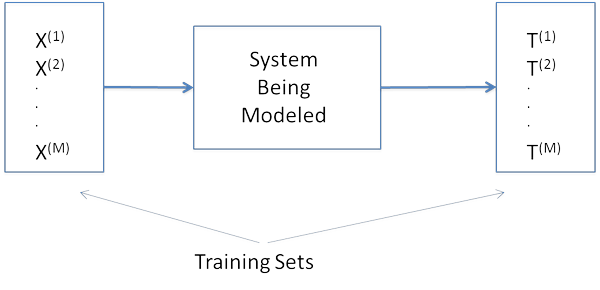Consider the system shown in Figure supervisedLearning. We assume that we don’t know anything about the internal working of the system, i.e., it exists as a black box. The only information we have about the system is that when it is subject to an input vector $X{(m)}=(x_1{(m)},...,x_N{(m)}), 1 \leq m \leq M$ belonging to a Training Set, then it results in the vector $T{(m)}=(t_1{(m)},...,t_K{(m)}), 1 \leq m \leq M$. The vector $T{(m)}$ is called the "Ground Truth", and corresponds to the "correct" output for the input $X{(m)}$.

In :
#Label
nb_setup.images_hconcat(["DL_images/Label.png"], width=600)

Out: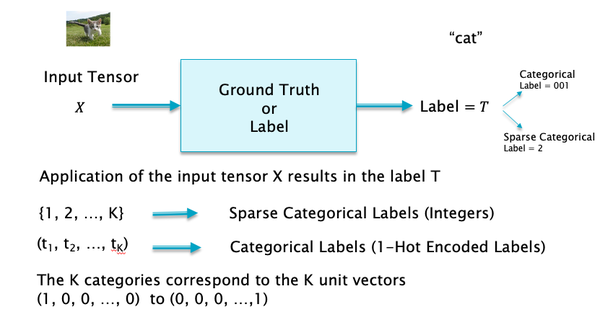The label vector $T(m)$ can be defined in two ways, as shown in Figure Label:

1. Sparse Categorical Labels: In this case the label is simply an integer corresponding to the category that the object belongs to.

2. Categorical Labels: The label is defined as a 1-Hot vector $(t_1(m),...,t_K(m))$, such that if the input vector $X{(m)}$ belongs to the category $q$, then $t_q{(m)} = 1$ and $t_k{(m)} = 0, k\neq q$

In :
#SL2
nb_setup.images_hconcat(["DL_images/SL2.png"], width=600)

Out: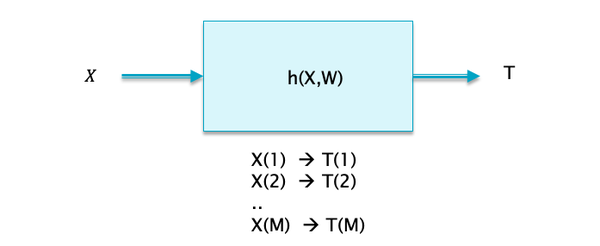The Supervised Learning problem is defined as the process of synthesizing a model for the system based on the labeled training pairs. This model should be such that if subject it to an input $X$ (which is not one of the $M$ training vectors), then it should result in a “correct” output $T$ (see Figure SL2). The model is a mathematical function $h(X,W)$, where $W$ is the set of model parameters. Supervised Learning the process of finding these parameters such that the model gives correct responses to the new input data that is not part of the training dataset.

The solution to the Supervised Learning problem proceeds in three steps:

1. The problem is recast using the framework of Statistical Estimation theory. This enables the formulation of the problem as an optimization problem.

2. A parametrized model $h(X,W)$ is proposed for the system. The structure of the model is usually a creative breakthrough inspired by experimental results and/or intuitive insights. In the case of DLNs it has taken inspiration from what we understand about the workings of biological brains.

3. The parameters of the model in step (2) are estimated by solving the optimization problem from step (1).

## Statistical Classification Formulation¶

In :
#SL3
nb_setup.images_hconcat(["DL_images/SL3.png"], width=600)

Out: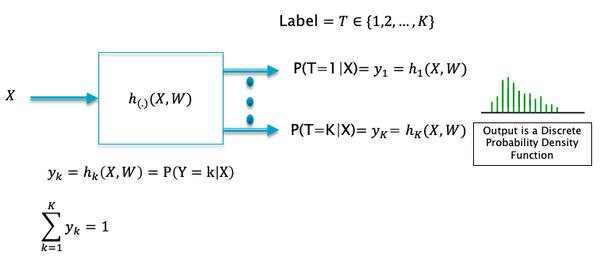If we insist that the output of the model be a 1-hot vector, i.e., input data is classified into one of the K categories with 100% certainity, then in general the Supervised Learning problem does not have a solution. Instead we resort to Statistical Classification (see Figure SL3), such that the model outputs probabilities of the input X belonging to the various categories. We use the notation $y = (y_1,...,y_K)$ for these probabilities, and since the output is guaranteed to be in one of the K categories, it follows that $$\sum_{k=1}^K y_k = 1$$

These probabilities are defined as the following conditional probabilities:

$$y_k = P(T = k|X),\ \ 1\le k\le K$$

Hence we expect the model output $Y$ to be a vector of conditional probabilities, such that the $k^{th}$ component of $Y$ is given by the conditional probability that the input vector $X$ belongs to the category $k$. We are asking the model to give us the conditional probabilities for the input $X$ to belong to a particular class, as opposed to the actual system which outputs the Ground Truth with full certainity (see Figure supervisedLearning). This formulation allows us to apply tools from Maximum Likelihood Estimation to the problem, which we do next.

Using the categorical labels formulation:

$$P(T=(1,0,...,0)|X) = y_1$$$$P(T=(0,1,,...,0)|X) = y_2$$

. . . $$P(T=(0,0,,...,1)|X) = y_K$$

This set of equations can be written compactly as:

$$P(T=(t_1,...,t_K)|X) = (y_1)^{t_1} y_2^{t_2}\ ...\ y_K^{t_K}$$

Using Maximum Likelihood Estimation Theory, the Likelihood function for this problem is given by product over all the samples in the training dataset. Re-introducing the superscript $m$ for the $m^{th}$ traning sample, we get the following expression for the Likelihood Function $l$:

$$l = \prod_{m=1}^M P(T{(m)}|X{(m)}) = \prod_{m=1}^M \prod_{k=1}^K (y_k{(m)})^{t_k{(m)}}$$

Taking the natural log on both sides, the Log Likelihood is given by

$$L = -\log l = -{1\over M}\sum_{m=1}^M \sum_{k=1}^K t_k{(m)}\log y_k{(m)}$$

Note the following: (1) Since $0\le y_k{(m)}\le 1$, the negative sign ensures that the expression for $L$ stays positive, and (2) We divide by $M$ to average out the contribution from each training sample.

In :
#supervisedLearningSolution
nb_setup.images_hconcat(["DL_images/supervisedLearningSolution.png"], width=600)

Out: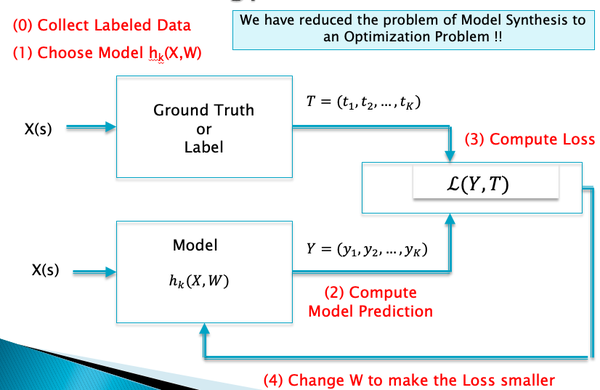$L(W)$ is referred to as the Loss Function (sometimes also called the Error Function) and it measures how close the model output $Y$ are to the desired outputs $T$. Hence the end result of this theory is quite intuitive: It says that the best classification model is the one that minimizes the distance (as measured by the Cross Entropy function), between the model output and the training label. In a following section we will show that best regression model also has a similar interpretation, it is the model that minimizes the Mean Square Error distance between the model output and the training data.

Figure supervisedLearningSolution illustrates the solution to the Supervised Learning problem. The top half of the figure shows the system that is being modeled: The output $T{(m)}$ of the system being the Ground Truth corresponding to the input $X{(m)}$. The bottom half of the figure shows a DLN model $h(X,W)$ for this system. When we subject the model to the input $X{(m)}$, it results in output vector $Y(m) = (y_1)m),...,y_K(m))$ for $1 \leq m \leq M$ and the corresponding per sample loss $\mathcal{L}(m)$ (defined below). The training process consists of tweaking the values of $W$ such that loss gradually decreases.

## The Cross Entropy Loss Function¶

Note that the expression for the Loss Function $L$ can be written as

$$L = {1\over M}\sum_{m=1}^M \mathcal{L}{(m)}$$

where

$$\mathcal{L}{(m)} = -\sum_{k=1}^K t_k{(m)}\log y_k{(m)}$$

$\mathcal{L}(m)$ can be interpreted as the Loss Function for the $m^{th}$ training sample $(X(m), T(m))$, such that the Loss $L$ for the entire training dataset is the average of the per sample losses.

The function $\mathcal{L}$ is known as the Cross Entropy function, and is well known from the field of Information Theory, where it is used as a measure of the distance between probability distributions. The above expression says that the overall Loss Function $L$ is obtained by averaging out the per sample Cross Entropy Loss between the distributions $T{(m)}=(t_1{(m)},...,t_K{(m)})$ and $Y{(m)}=(y_1{(m)},...,y_K{(m)})$.

In :
#SL4
nb_setup.images_hconcat(["DL_images/SL4.png"], width=600)

Out: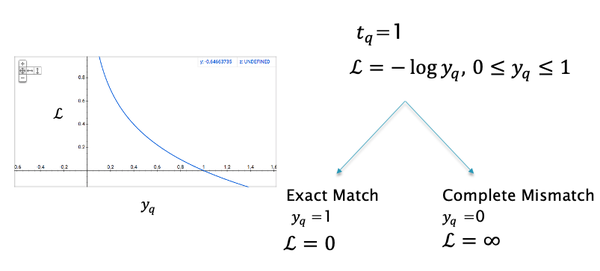Note that if $t_q = 1$, then $\mathcal L$ can be written as

$$\mathcal L = -\log y_q$$

From this expression it is easy to see that if the two distributions match (i.e. the model's prediction matches the ground truth with $y_q = 1$), then $\mathcal L = 0$, while if there is mismatch, then the value of $\mathcal L$ rises steeply, and approaches infinity for a perfect mismatch (thus justifying its interpretation as a measure of the mis-match). The value of $\mathcal {L}$ can be plotted in full, as shown in Figure SL4.

There is an important special case for the Cross Entropy Loss for K = 2, which is the Binary Cross Entropy Loss:

$$\mathcal L = -[t\log y + (1-t)\log (1-y)]$$

From this expression we can see that for case K = 2, it is sufficient for the classification system to have a single output $y$, since the other output is always $1-y$.

## The Regression Problem¶

In :
#SL5
nb_setup.images_hconcat(["DL_images/SL5.png"], width=600)

Out: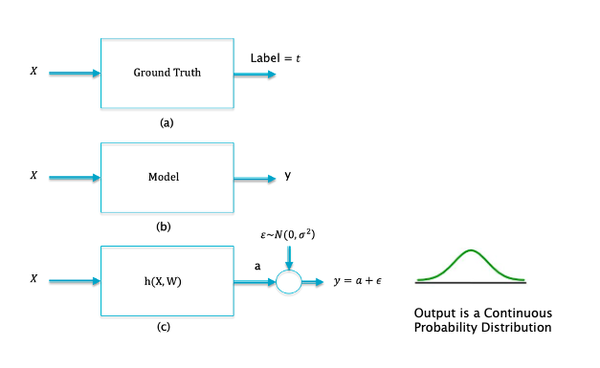The Regression Problem is defined as follows (see Part (a) of Figure SL5): We are given M training pairs of inputs and outputs $(X(m),t(m)), m = 1,2,…,M$ (assume that X is a vector and and the label t is a scalar, this can be easily extended to the case of vector valued labels). As shown in Part (b) of the figure, our objective is to find a Model whose output y is required to give “correct” values of the label t, even for values of X that are not part of the training set. Hence in this case the system is used to predict a continuous valued label (as opposed to a discrete output when doing classification).

When doing classification, we did not attempt to predict the label with 100% certainity, but instead resorted to a statistical methodology in which we used a Neural Network model to estimate the (discrete) distribution $P(T=k|X) = y_k, k = 1,...,K$ of the model output falling into the $K$ classes. Similarly in regression, we won't try to predict the exact ground truth t (which is now a real number), but instead have the model provide a distribution of estimated output values $y$. However unlike in classification, we assume that the distribution of y is known, i.e., it is Normal, and then use a Neural Network to compute the Mean of this Normal distribution. Proceeding along these lines, we assume that the output $y$ can be modeled as follows:

$$y = h(X,W) + \epsilon$$

where $h(X,W)$ is a Neural Network with weight parameters W that have to be estimated from the training data (see Part (c) of the figure) and $\epsilon$ is a Normally distributed random variable to mean 0 and variance $\sigma$ (which is also an unknown parameter). From this equation the probability density for $y$ is given by

$$f(y|X,W) = {1\over{\sqrt{2\pi\sigma^2}}}\exp(-{{(y-h(X,W))^2}\over{2\sigma^2}})$$

We will shortly show that the "goodness" of this model is a function of how close the mean of this distribution $h(X,W)$ is to the label t, using the Mean Square Error as a measure of distance.

As before we proceed using the Maximum Likelihood methodology in order to find the best parameters $W$ for the model. The best parameters are those that maximize the probability of occurance of the training data $(X(1),t(1)),...,(X(M),t(M)))$, which is quantlified by the Likelihood Function $l$ given by

$$l = \prod_{m=1}^M {1\over{\sqrt{2\pi\sigma^2}}}\exp(-{{(t(m) - h(X(m),W))^2}\over{2\sigma^2}})$$

and the Log Likelihood L is given by (dividing by M for normalization, and using the negative sign to ensure that the values of L stay positive)

$$L = -{\log l\over M} = {1\over 2}\log {2\pi\sigma^2} + {1\over M}\sum_{m=1}^M {{(t(m) - h(X(m),W))^2}\over{2\sigma^2}}$$

Assuming that the value of $\sigma$ is fixed, the effective Log Likelihood is given by

$$L = {1\over M}\sum_{m=1}^M (t(m) - h(X(m),W))^2$$

Hence the best model parameters $W$ are those that minimize the Mean Square Error of the difference between the model output $h(X,W)$ and the labels $t$. The MSE Loss for a single sample is given by

$$\mathcal L = (t-h(X,W))^2$$

This formula can be generalized for the case when there are $K$ outputs to

$$\mathcal L = {1\over K}\sum_{k=1}^K (t_k - h_k(X,W))^2$$

Another popular Loss Function used in Regression Problems is the Mean Absolute Error (MAE), given by

$$\mathcal L = {1\over K}\sum_{k=1}^K |t_k - h_k(X,W)|$$

## Summary¶

In summary, the process of minimizing the Loss Function $\mathcal L$ and thereby finding the best set of parameters $W$ (also called the Training Algorithm), is carried out in the following steps:

1. Given an input $X$, compute the model output $h(X,W)$.

2. Use $h(X,W)$ and the Ground Truth $T$ to compute the Cross Entropy Loss Function $\mathcal L$.

3. Tweak the parameters $W$ so that $\mathcal L$ value decreases

4. Repeat steps 1 to 3, until the Loss Function $\mathcal L$ drops below some threshold.

Given a family of models parametrized by W, we have reduced the problem of finding the best model to one of solving an optimization problem. A lot of the effort in Supervised Learning research has gone into finding efficient ways for solving this optimization problem, which can become non-trivial when the model contains millions of parameters. Since the 1980s, the most popular technique for solving this problem is the Backprop algorithm which is an efficient way to implement the classical Gradient Descent optimization algorithm in the DLN context. Backprop works by iteratively processing each training set pair $(X,T)$ and using this information to make incremental changes to the model parameter estimates in a fashion that causes the Loss Function to decrease.

As promised we have shown how to estimate the model parameters by using the training dataset. However this solves only half the problem, since we still haven't revealed $h(X,W)$ the functional form of the model. The rest of the monograph is devoted to addressing this. We start off with simple Linear Models in Chapter LinearLearningModels, and then move on to Deep Learning Models in Chapter NNDeepLearning. Convolutional Neural Networks are discussed in Chapter ConvNets. These models work especially well for classifying images. Recurrent Neural Networks are discussed in Chapter RNNs. These models are well suited for detecting patterns in sequences of data, such those that arise in NLP applications.